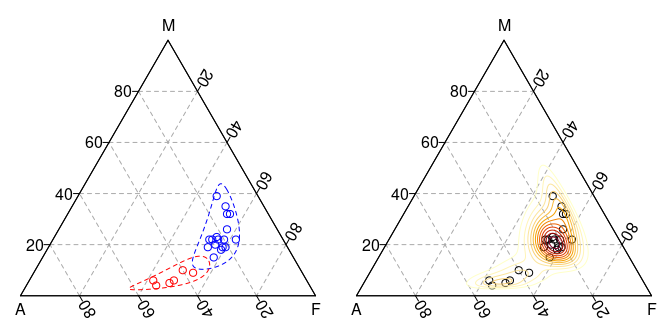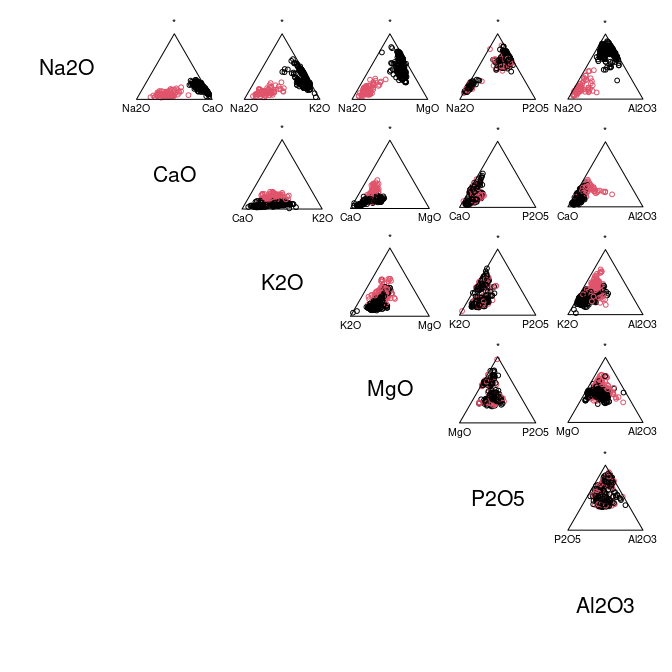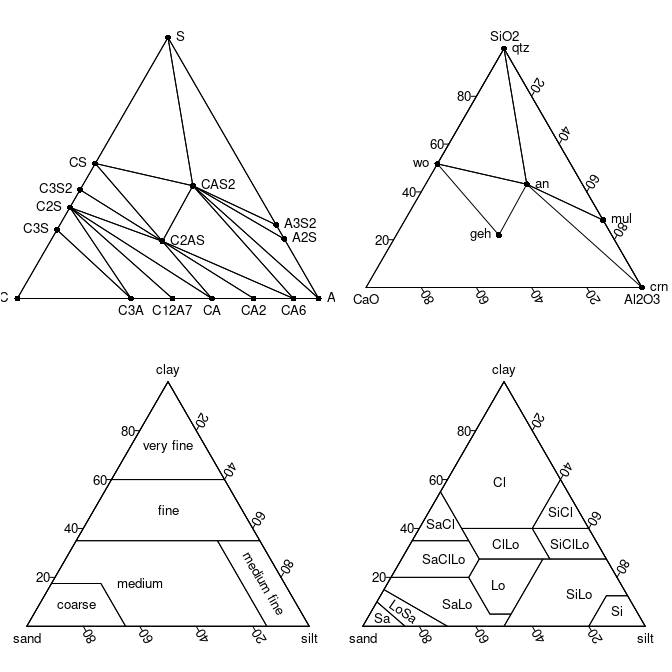## Overview

Ternary plots made simple. isopleuros allows to create ternary plot using graphics. It provides functions to display the data in the ternary space, to add or tune graphical elements and to display statistical summaries. It also includes common ternary diagrams useful for the archaeologist (e.g. soil texture charts, ceramic phase diagram).

isopleuros is a dependency-free package designed to be as simple as possible. If you need finer tuning or more advanced features, you should consider the Ternary or ggtern package.

## Installation

You can install the released version of isopleuros from CRAN with:

install.packages("isopleuros")

And the development version from GitHub with:

# install.packages("remotes")
remotes::install_github("tesselle/isopleuros")

## Usage

## Load package
library(isopleuros)

isopleuros tries to mimic the way graphics works as much as possible.

## Install extra package (if needed)
# install.packages("folio")

## Data from Barrera and Velde 1989
data("verre", package = "folio")

## Select data
coda <- verre[, c("Na2O", "CaO", "K2O")]

## Ternary plot
ternary_plot(coda, panel.first = ternary_grid())

## Split data
groups <- split(coda, f = coda$Na2O > 5) ## Add tolerance ellipses for (group in groups) { ternary_tolerance(group, level = 0.975, border = "blue", lty = 2) }## Select data coda <- verre[, c("Na2O", "CaO", "K2O", "MgO", "P2O5", "Al2O3")] ## Ternary plots with marginal compositions ternary_pairs(coda, col = as.factor(coda$Na2O > 5))par(mfrow = c(2, 2), mar = c(0, 0, 0, 0) + 0.1)

## Ceramic phase diagram
ternary_plot(NULL, axes = FALSE, ann = FALSE, frame.plot = TRUE)
triangle_phase_cas(symbol = TRUE, pch = 16)

ternary_plot(NULL, xlab = "CaO", ylab = "Al2O3", zlab = "SiO2")
triangle_phase_ceramic(symbol = TRUE, pch = 16)

## HYPRES soil texture
ternary_plot(NULL, xlab = "sand", ylab = "silt", zlab = "clay")
triangle_soil_hypres()

## USDA (1951) soil texture
ternary_plot(NULL, xlab = "sand", ylab = "silt", zlab = "clay")
triangle_soil_usda(symbol = TRUE)## Contributing

Please note that the isopleuros project is released with a Contributor Code of Conduct. By contributing to this project, you agree to abide by its terms.